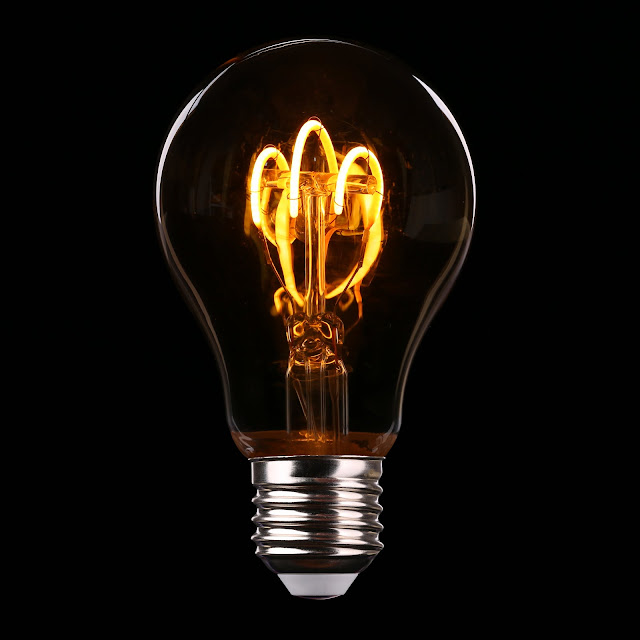What is Electricity? - Electrical C & V

# Electrical C & V

electrical engineering information, basic electrical, electrical laws, machines, transformer, motors, types of motor, induction motor, dc motor, servo motor, synchronous motor, dynamo or generator, power system, transmission line, mcc, generation station, electronics, devices, diodes, sensors, circuit breaker, miniature circuit breaker, air circuit breaker, vaccum circuit breaker, sf6 circuit breaker, variable frequency drive

## 18 February 2020

Electricity

Thousands of years ago, around 600 BC In Greece Thales, a scientist in Greece, found that when a substance called amber is rubbed with a wool cloth, it has the property of attracting small objects towards it. The property due to which substances are electrically is called 'electricity'. When the charge flows in a wire or conductor, it is called current electricity. There are two types of charge - positive charge (+ ve charge) and negative charge (-ve charge).Conductors & Non-Conductors

The materials through which the electric charge flows easily are called conductors and the substances through which the charge does not flow, are called nonconductors. Almost all metals, acid bases, aqueous solutions of salts, human body, etc. are examples of electrical conductors and wood, rubber, paper, mica, etc. are examples of dielectric materials.

Electric Current

The flow of charge is called Electric Current. The flow of charge in solid conductors is caused by the transfer of electrons from one place to another. Whereas this flow is caused by the movement of ions in fluids such as acids, bases, and salts, in aqueous solutions and gases. If the current in a circuit flows in one direction, it is called Direct current, and if the direction of the current changes continuously, it is called 'Alternating current'.Potential Difference

The work done in moving the conductor from one end to another by a single charge is called the difference between the two ends.

v = w / q (where v = potential difference, w = work and q = flowing charge.
The unit of difference is volt.

Electric Cell

The chemical energy is converted into electrical energy by various chemical reactions in the electrical cell. The electric cell consists of two metal rods called electrodes.
Power cells are mainly of two types -

1. Primary cell - chemical energy is directly converted into electrical energy. Examples are Voltaic Cell, Leclanche Cell, Daniel Cell, Bunsen Cell etc.

2. Secondary cell - In this first electrical energy is converted into chemical energy, then chemical energy into electrical energy. It is used to start motorcars, trucks etc.

Coulomb's Law

According to Coulomb's, the force between two stationary charges is proportional to the product of their quantities and inversely proportional to the square of the distance between them.
F = k (Q1Q2 / R2)
Q1, Q2 Two point charges are located at R distance from each other.

MORE DETAILS:-

Parts of Electric:-